Theory and Modern Applications

# An efficient new perturbative Laplace method for space-time fractional telegraph equations

## Abstract

In this paper, we propose a new technique for solving space-time fractional telegraph equations. This method is based on perturbation theory and the Laplace transformation. Fractional Taylor series and fractional initial conditions have been introduced. However, all the previous works avoid the term of fractional initial conditions in the space-time telegraph equations. The results of introducing fractional order initial conditions and the Laplace transform for the studied cases show the high accuracy, simplicity and efficiency of the approach.

## 1 Introduction

Telegraph equations are hyperbolic partial differential equations that are applicable in several fields such as wave propagation , signal analysis , random walk theory , etc. In recent years, there has been a great deal of interest in fractional differential equations [4, 5]. Time-fractional telegraph equations have been studied by Orsingher and Zhao  and Orsingher and Beghin . Telegraph equations apply to high-frequency transmission lines such as telegraph wires and radio frequency conductors. They are also applicable to designing high-voltage transmission lines.

In this paper, we consider two different types of telegraph equations. The first one is the space-fractional telegraph equation

$\frac{{\partial }^{\alpha }u}{\partial {x}^{\alpha }}=\frac{{\partial }^{2}u}{\partial {t}^{2}}+a\frac{\partial u}{\partial t}+bu+f\left(x,t\right),\phantom{\rule{1em}{0ex}}0<\alpha \le 2,$

subject to the initial and boundary conditions

$u\left(0,t\right)=\phi \left(t\right),\phantom{\rule{2em}{0ex}}{u}_{x}\left(0,t\right)=\psi \left(t\right),\phantom{\rule{2em}{0ex}}u\left(x,0\right)=\varphi \left(x\right),\phantom{\rule{1em}{0ex}}0

and the second equation is the classical time-fractional telegraph equation

$\frac{{\partial }^{\alpha }u}{\partial {t}^{\alpha }}+\frac{{\partial }^{\alpha -1}u}{\partial {t}^{\alpha -1}}+u=\frac{{\partial }^{2}u}{\partial {x}^{2}}+f\left(x,t\right),\phantom{\rule{1em}{0ex}}1<\alpha \le 2,$

subject to the initial conditions

$u\left(x,0\right)=\phi \left(t\right),\phantom{\rule{2em}{0ex}}{u}_{t}\left(x,0\right)=\psi \left(x\right),$

where u can be considered as a function depending on distance (x) and time (t), a and b are constants depending on a given problem and f, φ, ϕ, ψ are known continuous functions. The second equation has been solved by Das et al.  using the homotopy analysis method.

The aim of this paper is to introduce a new method for fractional space-time telegraph equations. This new technique is a combined form of the perturbation method  with the Laplace transform. This method is called the perturbation Laplace method (PLM). Moreover, we have introduced fractional order initial conditions for space-time telegraph equations. Point to be noted regarding fractional differential equations is that one should use fractional Taylor series. To make the calculation easy and simple, for the first time, we have used the Laplace transform to solve the systems of equations formed after applying homotopy perturbation instead of applying an inverse operator. Through the Laplace transform of fractional order term, it is easy to judge that one must use fractional order initial conditions. It is easy to judge, by applying the Laplace transformation, that it is essential to use a fractional order initial condition to analyze any physical phenomenon which has been expressed in terms of fractional differential equations. To the best of authors’ knowledge, in the literature on space-fractional telegraph equations , there is no closed form solution for different values of α except for the standard case, i.e., for $\alpha =2$. The elegance of this article can be attributed to its endeavor of finding the solution in a simple way by considering only the PLM. Two examples which show that only a few iterations are needed to obtain accurate approximate solutions are solved.

## 2 Fractional calculus theory

We give some basic definitions and properties of the fractional calculus theory proposed by Jumarie  which are used further in this paper.

Definition 1 Let $f:R\to R$, $x\to f\left(x\right)$, denote a continuous (but not necessarily differentiable) function. Then its fractional derivative of order α, $\alpha <0$, is defined by the following expression:

${f}^{\left(\alpha \right)}\left(x\right)=\frac{1}{\mathrm{\Gamma }\left(-\alpha \right)}{\int }_{0}^{x}{\left(x-\xi \right)}^{-\alpha -1}f\left(\xi \right)\phantom{\rule{0.2em}{0ex}}d\xi .$

For positive α, we define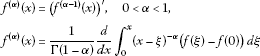and

${f}^{\left(\alpha \right)}\left(x\right)={\left({f}^{\left(\alpha -n\right)}\left(x\right)\right)}^{\left(n\right)},\phantom{\rule{1em}{0ex}}n\le \alpha

With this definition, the Laplace transform $L\left\{\cdot \right\}$ of the fractional derivative is defined as follows:

$L\left\{{f}^{\left(\alpha \right)}\left(x\right)\right\}={s}^{\alpha }L\left\{f\left(x\right)\right\}-{s}^{\alpha -1}f\left(0\right),\phantom{\rule{1em}{0ex}}0<\alpha <1.$

Proposition 1 (On the decomposition of fractional derivatives)

Let α be such that $0<3\alpha <1$. There are two different ways to obtain ${D}^{3\alpha }f\left(x\right)$ (see ). One can calculate ${D}^{\alpha }{D}^{\alpha }{D}^{\alpha }f\left(x\right)$ to obtain the Laplace transform

$L\left\{{D}^{\alpha }{D}^{\alpha }{D}^{\alpha }f\left(x\right)\right\}={s}^{3\alpha }f\left(s\right)-{s}^{3\alpha -1}f\left(0\right)-{s}^{2\alpha -1}{f}^{\left(\alpha \right)}\left(0\right)-{s}^{\alpha -1}{f}^{\left(2\alpha \right)}\left(0\right).$
(1)

Proposition 2 Assume that the continuous function $f:R\to R$, $x\to f\left(x\right)$ has a fractional derivative of order for any positive integer k and any α, $0<\alpha \le 1$. Then the following equality holds:

$f\left(x+h\right)=\sum _{k=0}^{\mathrm{\infty }}\frac{{h}^{\alpha k}}{\alpha k!}{f}^{\left(\alpha k\right)}\left(x\right),\phantom{\rule{1em}{0ex}}0<\alpha \le 1.$

On making $x=0$ and the substitution $h\to x$, we obtain the fractional Maclaurin series

$f\left(x\right)=\sum _{k=0}^{\mathrm{\infty }}\frac{{x}^{\alpha k}}{\alpha k!}{f}^{\left(\alpha k\right)}\left(0\right),\phantom{\rule{1em}{0ex}}0<\alpha \le 1.$

## 3 Perturbative Laplace method

In order to elucidate the solution procedure of the perturbative Laplace method (PLM), we consider the following fractional differential equation: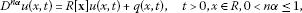(2)

where ${D}^{n\alpha }=\frac{{\partial }^{n\alpha }}{\partial {t}^{n\alpha }}$,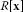is generally the linear differential operator with respect to the variable x and $f\left(x\right)$, $q\left(x,t\right)$ are continuous functions. In view of HPM , we can construct a homotopy for Eq. (2) as follows: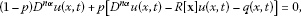(3)

or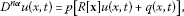(4)

where $p\in \left[0,1\right]$ is an embedding parameter. If $p=0$, Eq. (3) and Eq. (4) become

${D}^{n\alpha }u\left(x,t\right)=0,$
(5)

and when $p=1$, both Eq. (3) and Eq. (4) turn out to be the original fractional differential equation (2).

The homotopy perturbation method  admits a solution in the form

$u={p}^{0}{u}_{0}+{p}^{1}{u}_{1}+{p}^{2}{u}_{2}+\cdots .$
(6)

Setting $p=1$ in the solution of Eq. (6), we get

$u={u}_{0}+{u}_{1}+{u}_{2}+\cdots .$
(7)

Invoking Eq. (6) into Eq. (4) and collecting the terms with the same powers of p, we can obtain a series of equations of the following form: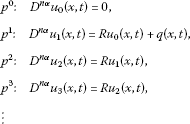(8)

By using the definition given in Eq. (1), we get

$\begin{array}{r}{p}^{0}:\phantom{\rule{1em}{0ex}}{s}^{n\alpha }{u}_{0}\left(x,s\right)-{s}^{n\alpha -1}{u}_{0}\left(x,0\right)-{s}^{\left(n-1\right)\alpha -1}{u}_{0}^{\left(\alpha \right)}\left(x,0\right)\\ \phantom{{p}^{0}:\phantom{\rule{1em}{0ex}}\phantom{\rule{1em}{0ex}}}-{s}^{\left(n-2\right)\alpha -1}{u}_{0}^{\left(2\alpha \right)}\left(x,0\right)-\cdots -{s}^{\alpha -1}{u}_{0}^{\left(\left(n-1\right)\alpha \right)}\left(x,0\right)=0,\\ {p}^{1}:\phantom{\rule{1em}{0ex}}{s}^{n\alpha }{u}_{1}\left(x,s\right)=R{u}_{0}\left(x,s\right)+q\left(x,s\right),\\ {p}^{2}:\phantom{\rule{1em}{0ex}}{s}^{n\alpha }{u}_{2}\left(x,s\right)=R{u}_{1}\left(x,s\right),\\ {p}^{3}:\phantom{\rule{1em}{0ex}}{s}^{n\alpha }{u}_{3}\left(x,s\right)=R{u}_{2}\left(x,s\right),\\ ⋮\end{array}$
(9)

Solving Eq. (9) for ${u}_{0},{u}_{1},{u}_{2},{u}_{3},\dots$ respectively, by using the fractional initial value conditions, we get

$\begin{array}{r}{p}^{0}:\phantom{\rule{1em}{0ex}}{u}_{0}\left(x,t\right)={L}^{-1}\left\{\frac{1}{{s}^{n\alpha }}\left({s}^{n\alpha -1}{u}_{0}\left(x,0\right)+{s}^{\left(n-1\right)\alpha -1}{u}_{0}^{\left(\alpha \right)}\left(x,0\right)\\ \phantom{{p}^{0}:\phantom{\rule{1em}{0ex}}\phantom{\rule{1em}{0ex}}}+{s}^{\left(n-2\right)\alpha -1}{u}_{0}^{\left(2\alpha \right)}\left(x,0\right)+\cdots +{s}^{\alpha -1}{u}_{0}^{\left(n\alpha \right)}\left(x,0\right)\right)\right\},\\ {p}^{1}:\phantom{\rule{1em}{0ex}}{u}_{1}\left(x,t\right)={L}^{-1}\left\{\frac{1}{{s}^{n\alpha }}\left(R{u}_{0}\left(x,s\right)+q\left(x,s\right)\right)\right\},\\ {p}^{2}:\phantom{\rule{1em}{0ex}}{u}_{2}\left(x,t\right)={L}^{-1}\left\{\frac{1}{{s}^{n\alpha }}\left(R{u}_{1}\left(x,s\right)\right)\right\},\\ {p}^{3}:\phantom{\rule{1em}{0ex}}{u}_{3}\left(x,t\right)={L}^{-1}\left\{\frac{1}{{s}^{n\alpha }}\left(R{u}_{2}\left(x,s\right)\right)\right\},\\ ⋮\end{array}$
(10)

Substituting successive iterations in Eq. (7) will give the required result.

## 4 Space-time fractional telegraph equations

Example 1 Let us consider the space-fractional telegraph equation

$\frac{{\partial }^{\alpha }u}{\partial {x}^{\alpha }}=\frac{{\partial }^{2}u}{\partial {t}^{2}}+\frac{\partial u}{\partial t}+u,\phantom{\rule{1em}{0ex}}0<\alpha \le 2,$
(11)

subject to the initial and boundary conditions

$u\left(0,t\right)={e}^{-t},\phantom{\rule{2em}{0ex}}{u}_{x}\left(0,t\right)={e}^{-t},\phantom{\rule{2em}{0ex}}u\left(x,0\right)={e}^{x},\phantom{\rule{1em}{0ex}}0

In order to illustrate the efficiency of our method, we replace the fractional order α, $0<\alpha \le 2$, by the order 2α, $0<\alpha \le 1$, in Eq. (11)

$\frac{{\partial }^{2\alpha }u}{\partial {x}^{2\alpha }}=\frac{{\partial }^{2}u}{\partial {t}^{2}}+\frac{\partial u}{\partial t}+u,\phantom{\rule{1em}{0ex}}0<\alpha \le 1,$
(12)

subject to the initial and boundary conditions

$u\left(0,t\right)={e}^{-t},\phantom{\rule{2em}{0ex}}{u}^{\left(\alpha \right)}\left(0,t\right)={e}^{-t},\phantom{\rule{2em}{0ex}}u\left(x,0\right)={e}^{x},\phantom{\rule{1em}{0ex}}0

Using the procedure in Section 2, we can write Eq. (12) in the form of recurrence equations as follows: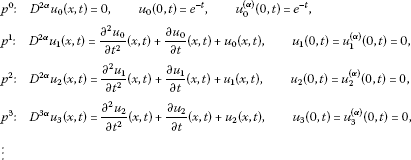(13)

In view of Eq. (9), Eq. (13) can be written in the following form: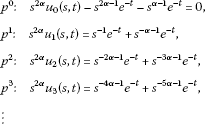(14)

Solving Eq. (14) for ${u}_{0},{u}_{1},{u}_{2},{u}_{3},\dots$ , we get the following form: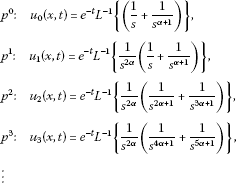(15)

Equation (15) in the most refined form can be written as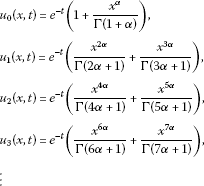(16)

The solution in a series form can be expressed as

$\begin{array}{rcl}u\left(x,t\right)& =& {e}^{-t}\left(1+\frac{{x}^{\alpha }}{\mathrm{\Gamma }\left(1+\alpha \right)}+\frac{{x}^{2\alpha }}{\mathrm{\Gamma }\left(2\alpha +1\right)}+\frac{{x}^{3\alpha }}{\mathrm{\Gamma }\left(3\alpha +1\right)}+\frac{{x}^{4\alpha }}{\mathrm{\Gamma }\left(4\alpha +1\right)}+\frac{{x}^{5\alpha }}{\mathrm{\Gamma }\left(5\alpha +1\right)}+\cdots \right)\\ =& \sum _{k=0}^{\mathrm{\infty }}\frac{{e}^{-t}{x}^{k\alpha }}{\mathrm{\Gamma }\left(1+k\alpha \right)}={e}^{-t}{E}_{\alpha }\left({x}^{\alpha }\right),\end{array}$

where ${E}_{\alpha }$ denotes the Mittag-Leffler function.

Example 2 We consider the nonhomogeneous fractional time telegraph equation

$\frac{{\partial }^{\alpha }u}{\partial {t}^{\alpha }}+\frac{{\partial }^{\alpha -1}u}{\partial {t}^{\alpha -1}}+u=\frac{{\partial }^{2}u}{\partial {x}^{2}}+f\left(x,t\right),\phantom{\rule{1em}{0ex}}1<\alpha \le 2,$
(17)

subject to the initial conditions

$u\left(x,0\right)=0,\phantom{\rule{2em}{0ex}}{u}_{t}\left(x,0\right)=0,$

with

$f\left(x,t\right)=\frac{{t}^{n}}{\mathrm{\Gamma }\left(n+1\right)}sinhx,$

and for the fractional initial condition

$\frac{{\partial }^{\alpha }u}{\partial {t}^{\alpha }}+\frac{{\partial }^{\alpha -1}u}{\partial {t}^{\alpha -1}}+u=\frac{{\partial }^{2}u}{\partial {x}^{2}}+f\left(x,t\right),\phantom{\rule{1em}{0ex}}1<\alpha \le 2,$
(18)

subject to the initial conditions

$u\left(x,0\right)=0,\phantom{\rule{2em}{0ex}}{u}^{\left(\alpha \right)}\left(x,0\right)=0,$

with

$f\left(x,t\right)=\frac{{t}^{n}}{\mathrm{\Gamma }\left(n+1\right)}sinhx.$

By applying the aforesaid method, we can write Eq. (18) in the form of recurrence equations as follows:

$\begin{array}{r}{p}^{0}:\phantom{\rule{1em}{0ex}}{D}^{\alpha }{u}_{0}\left(x,t\right)=0,\phantom{\rule{2em}{0ex}}{u}_{0}\left(x,0\right)=0,\phantom{\rule{2em}{0ex}}{u}_{0}^{\left(\alpha \right)}\left(x,0\right)=0,\\ {p}^{1}:\phantom{\rule{1em}{0ex}}{D}^{\alpha }{u}_{1}\left(x,t\right)=-{u}_{0}^{\left(\alpha -1\right)}\left(x,t\right)-{u}_{0}\left(x,t\right)+\frac{{\partial }^{2}{u}_{0}\left(x,t\right)}{\partial {x}^{2}}+f\left(x,t\right),\\ \phantom{{p}^{1}:\phantom{\rule{1em}{0ex}}}{u}_{1}\left(x,0\right)={u}_{1}^{\left(\alpha \right)}\left(x,0\right)=0,\\ {p}^{2}:\phantom{\rule{1em}{0ex}}{D}^{\alpha }{u}_{2}\left(x,t\right)=-{u}_{1}^{\left(\alpha -1\right)}\left(x,t\right)-{u}_{1}\left(x,t\right)+\frac{{\partial }^{2}{u}_{1}\left(x,t\right)}{\partial {x}^{2}},\\ \phantom{{p}^{2}:\phantom{\rule{1em}{0ex}}}{u}_{2}\left(x,0\right)={u}_{2}^{\left(\alpha \right)}\left(x,0\right)=0,\\ {p}^{3}:\phantom{\rule{1em}{0ex}}{D}^{\alpha }{u}_{3}\left(x,t\right)=-{u}_{2}^{\left(\alpha -1\right)}\left(x,t\right)-{u}_{2}\left(x,t\right)+\frac{{\partial }^{2}{u}_{2}\left(x,t\right)}{\partial {x}^{2}},\\ \phantom{{p}^{3}:\phantom{\rule{1em}{0ex}}}{u}_{3}\left(x,0\right)={u}_{3}^{\left(\alpha \right)}\left(x,0\right)=0,\\ ⋮\end{array}$
(19)

By using Eq. (9), we can write Eq. (19) in the following form: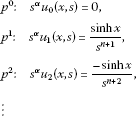Proceeding as before, we obtain

$\begin{array}{r}{p}^{0}:\phantom{\rule{1em}{0ex}}{u}_{0}\left(x,t\right)=0,\\ {p}^{1}:\phantom{\rule{1em}{0ex}}{u}_{1}\left(x,t\right)={L}^{-1}\left\{\frac{1}{{s}^{\alpha }}\left(\frac{sinhx}{{s}^{n+1}}\right)\right\},\\ {p}^{2}:\phantom{\rule{1em}{0ex}}{u}_{2}\left(x,t\right)={L}^{-1}\left\{\frac{1}{{s}^{\alpha }}\left(\frac{-sinhx}{{s}^{n+2}}\right)\right\},\\ ⋮\end{array}$
(20)

Equation (20) can also be written as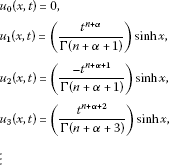and so on. In this way, the rest of components of the homotopy perturbation series can be obtained. Finally, we obtain the series solution as

$u\left(x,t\right)=\left(\frac{{t}^{n+\alpha }}{\mathrm{\Gamma }\left(n+\alpha +1\right)}-\frac{{t}^{n+\alpha +1}}{\mathrm{\Gamma }\left(n+\alpha +2\right)}+\frac{{t}^{n+\alpha +2}}{\mathrm{\Gamma }\left(n+\alpha +3\right)}+\cdots \right)sinhx.$

Figure 1 shows the approximate solution of Eq. (18) for different values of α using the perturbative Laplace method. The numerical result of the probability density function $u\left(x,t\right)$ for different fractional Brownian motions $\alpha =1.80,1.90,1.99$, and also for standard motion $\alpha =2$, is calculated for $n=1$, $x=2$ with respect to the variable t. The three-dimensional variation of $u\left(x,t\right)$ vs. x and t at $\alpha =1.5$ and $n=1$ is shown in Figure 2. It is seen from the figures that $u\left(x,t\right)$ increases with increasing t but decreases with increasing α, which assures the exponential decay of regular Brownian motion.

## 5 Conclusion

In this paper, we have introduced a combination of perturbation and Laplace methods for space-time fractional problem which we called the PLM. We described the method and used it in some fractional telegraph equations in order to show its applicability and validity. We achieved accurate approximations by using only a few numbers of iterations, which reveals efficiency of the new method. The solution very rapidly converges by utilizing the perturbation Laplace method. The PLM is also valid for other fractional differential equations, and this paper can be used as a standard paradigm for other applications.

## References

1. Weston VH, He S:Wave splitting of the telegraph equation in ${R}^{3}$ and its application to inverse scattering. Inverse Probl. 1993, 9: 789–812. 10.1088/0266-5611/9/6/013

2. Jordan PM, Puri A: Digital signal propagation in dispersive media. J. Appl. Phys. 1999, 85: 1273–1282. 10.1063/1.369258

3. Banasiak J, Mika R: Singular perturbed telegraph equations with applications in random walk theory. J. Appl. Math. Stoch. Anal. 1998, 11: 9–28. 10.1155/S1048953398000021

4. Oldham KB, Spanier J: The Fractional Calculus. Academic Press, New York; 1974.

5. Podlubny I: Fractional Differential Equations. Academic Press, New York; 1999.

6. Orsingher E, Zhao X: The space-fractional telegraph equation and the related fractional telegraph process. Chin. Ann. Math., Ser. B 2003, 24: 45–56. 10.1142/S0252959903000050

7. Orsingher E, Beghin L: Time-fractional telegraph equations and telegraph processes with Brownian time. Probab. Theory Relat. Fields 2004, 128: 141–160. 10.1007/s00440-003-0309-8

8. Das S, Vishal K, Gupta PK, Yildirim A: An approximate analytical solution of time-fractional telegraph equation. Appl. Math. Comput. 2011, 217: 7405–7411. 10.1016/j.amc.2011.02.030

9. He JH: Homotopy perturbation technique. Comput. Methods Appl. Mech. Eng. 1999, 178(3–4):257–262. 10.1016/S0045-7825(99)00018-3

10. Xu L: He’s homotopy perturbation method for a boundary layer equation in unbounded domain. Comput. Math. Appl. 2007, 54: 1067–1070. 10.1016/j.camwa.2006.12.052

11. Turkyilmazoglu M: Convergence of the homotopy perturbation method. Int. J. Nonlinear Sci. Numer. Simul. 2011, 12: 9–14.

12. Hetmaniok E, Nowak I, Slota D, Witula R: Application of the homotopy perturbation method for the solution of inverse heat conduction problem. Int. Commun. Heat Mass Transf. 2012, 39: 30–35. 10.1016/j.icheatmasstransfer.2011.09.005

13. Gupta PK, Singh M: Homotopy perturbation method for fractional Fornberg-Whitham equation. Comput. Math. Appl. 2011, 61: 250–254. 10.1016/j.camwa.2010.10.045

14. Golbabai A, Sayevand K: Analytical modelling of fractional advection-dispersion equation defined in a bounded space domain. Math. Comput. Model. 2011, 53: 1708–1718. 10.1016/j.mcm.2010.12.046

15. Momani S: Analytic and approximate solutions of the space- and time-fractional telegraph equations. Appl. Math. Comput. 2005, 170: 1126–1134. 10.1016/j.amc.2005.01.009

16. Jumarie G: Table of some basic fractional calculus formulae derived from a modified Riemann-Liouville derivative for non-differentiable functions. Appl. Math. Lett. 2009, 22: 378–385. 10.1016/j.aml.2008.06.003

## Acknowledgements

The second author is supported by Grant P201/11/0768 of the Czech Grant Agency (Prague). The fourth author is supported by Grant FEKT-S-11-2-921 of the Faculty of Electrical Engineering and Communication, Brno University of Technology.

## Author information

Authors

### Corresponding author

Correspondence to Yasir Khan.

### Competing interests

The authors declare that they have no competing interests.

### Authors’ contributions

The authors have made the same contribution. All authors read and approved the final manuscript.

## Authors’ original submitted files for images

Below are the links to the authors’ original submitted files for images.

## Rights and permissions

Open Access This article is distributed under the terms of the Creative Commons Attribution 2.0 International License (https://creativecommons.org/licenses/by/2.0), which permits unrestricted use, distribution, and reproduction in any medium, provided the original work is properly cited.

Reprints and Permissions

Khan, Y., Diblík, J., Faraz, N. et al. An efficient new perturbative Laplace method for space-time fractional telegraph equations. Adv Differ Equ 2012, 204 (2012). https://doi.org/10.1186/1687-1847-2012-204

• Accepted:

• Published:

• DOI: https://doi.org/10.1186/1687-1847-2012-204

### Keywords

• Fractional Order
• Fractional Derivative
• Fractional Differential Equation
• Fractional Brownian Motion
• Homotopy Analysis Method